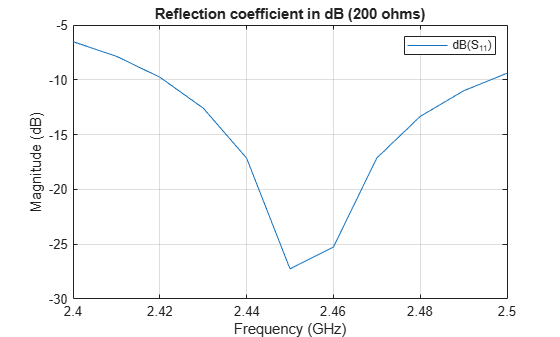# Sector Antenna for 2.4 GHz Wi-Fi™

This example models the inverted Amos sector antenna designed in . A sector antenna is a type of directional antenna with a sector-shaped radiation pattern. The word 'sector' is used here in the geometric sense as a portion of the circumference of a circle measured in degrees.

### Inverted Amos Sector Antenna

The antenna consists of a meander dipole (a Franklin antenna) backed by a reflector. The gain of the antenna depends on the number of vertically stacked dipoles in the meander. The current design will use 7 stacked dipoles as shown in Fig.5 in . All other dimensions follow .

```dipolearms = [88e-3 71e-3 73e-3 65e-3]; wirewidth = cylinder2strip(1e-3); notchL = 23.8e-3; notchW = 17e-3; spacing = 35.5e-3; GP_length = 660e-3; GP_Width = 75e-3;```

Paper  uses the dipole length of 84 mm with a 4 mm feeding gap. Antenna Toolbox uses the delta gap feed model. Add the feeding gap length to the length of the dipole resulting in the total length of 88 mm.

### Create Antenna

Create the inverted amos sector antenna.

```sector = sectorInvertedAmos('ArmWidth', wirewidth, 'ArmLength', dipolearms, ... 'NotchLength', notchL, 'NotchWidth', notchW,'Spacing', spacing, ... 'GroundPlaneLength', GP_length, 'GroundPlaneWidth', GP_Width); figure; show(sector); ```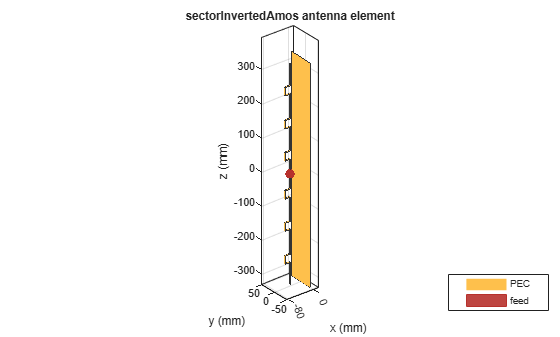### Mesh Structure

The antenna will operate between 2.4 GHz and 2.5 GHz. We manually mesh the structure by using at least 10 elements per wavelength at the highest frequency of the band.

```lambda = 3e8/2.5e9; figure; mesh(sector, 'MaxEdgeLength',lambda/10);```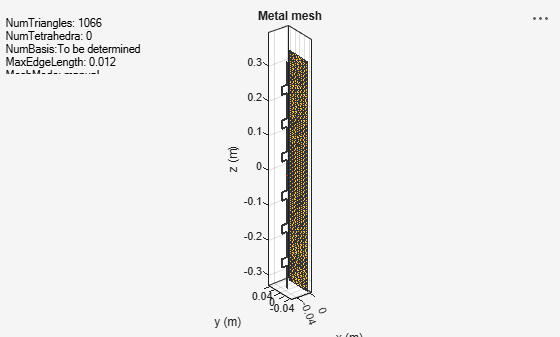### Radiation Pattern of Sector Antenna

The plots given below show the radiation patterns of the antenna at the center of the WiFi� band at 2.45 GHz. As the antenna name implies, the radiation pattern illuminated a sector with minimum radiation in the back lobe. The maximum directivity is 15.5 dBi, which is slightly higher than the 15.4 dBi reported in .

```figure; pattern(sector, 2.45e9); view(-45,30)```The plots below show the slices of the radiation patterns in the two principle planes.

```figure; pattern(sector, 2.45e9, 0, 1:1:360);```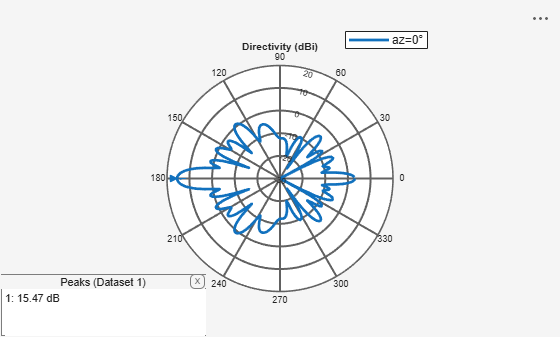```figure; pattern(sector, 2.45e9, 1:1:360,0);```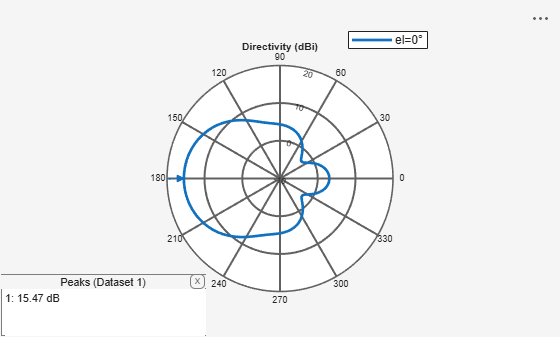### Antenna Performance Over the Wi-Fi Band

Measure the maximum directivity at an elevation angle of 90 degrees. It would be important to have a constant value over the entire band of interest. The plot below, displays the directivity at zenith from 2.4 GHz to 2.5 GHz.

```freq = linspace(2.4e9, 2.5e9, 11); D = zeros(1,numel(freq)); for m=1:numel(freq) D(m) = pattern(sector, freq(m), 180, 0); end figure; plot(freq./1e9, D); title('Directivity at el = 90'); ylabel('Directivity (dBi)'); xlabel('Frequency (GHz)'); grid on;```### Antenna Port Analysis

The plot given below shows the input impedance variation of the antenna over the band from 2.4 GHz to 2.5 GHz. The resistance varies between 100 to 400 ohms. To minimize reflections, the antenna is matched to a 200 ohm impedance.

```figure impedance(sector, freq);```Another way to study the port-based reflection characteristics is to plot the voltage standing wave ratio (VSWR) as well as the reflection coefficient measured with regard to the reference impedance of 200 ohms over the entire WiFi band. The antenna has VSWR around 2 or less and the reflection coefficient around -10 dB or less over the entire frequency band of interest. This indicates an acceptable matching over the WiFi band.

```figure vswr(sector, freq, 200);```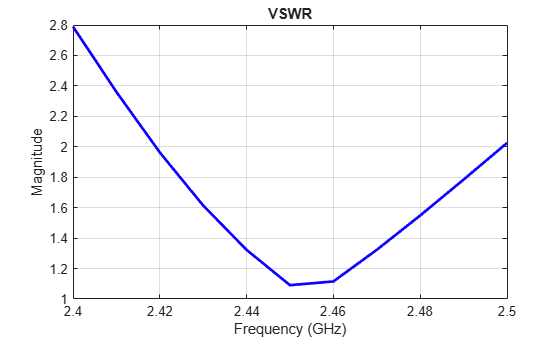Calculate the reflection coefficient using the S-parameter data.

```S = sparameters(sector, freq, 200); figure; rfplot(S); title('Reflection coefficient in dB (200 ohms)');```